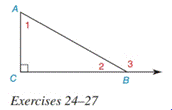Chapter 2.4, Problem 25EElementary Geometry For College St...

7th Edition
Alexander + 2 others
ISBN: 9781337614085

Solutions

Chapter
SectionElementary Geometry For College St...

7th Edition
Alexander + 2 others
ISBN: 9781337614085
Textbook ProblemIn Exercises 25 to 27 , see the figure for exercise 24 . Given: m ∠ 1 = x , m ∠ 2 = y , m ∠ 3 = 3 x Prove: x and y

To determine

To find:

The value of x and y.

Explanation

Given:

m1=x,

m2=y

And,

m3=3x

Figure (1)

Concept:

(1) Sum of acute angles in a right triangle is equal to 90°.

(2) Sum of linear pair of angles is equal to 180°.

Calculation:

Equate the sum of acute angles in a right triangle to 90°.

m1+m2=90°(1)

Substitute x for m1, and y for m2 in equation (1).

x+y=90°

Equate the sum of linear pair of angles to 180°

Still sussing out bartleby?

Check out a sample textbook solution.

See a sample solution

The Solution to Your Study Problems

Bartleby provides explanations to thousands of textbook problems written by our experts, many with advanced degrees!

Get Started

Find more solutions based on key concepts